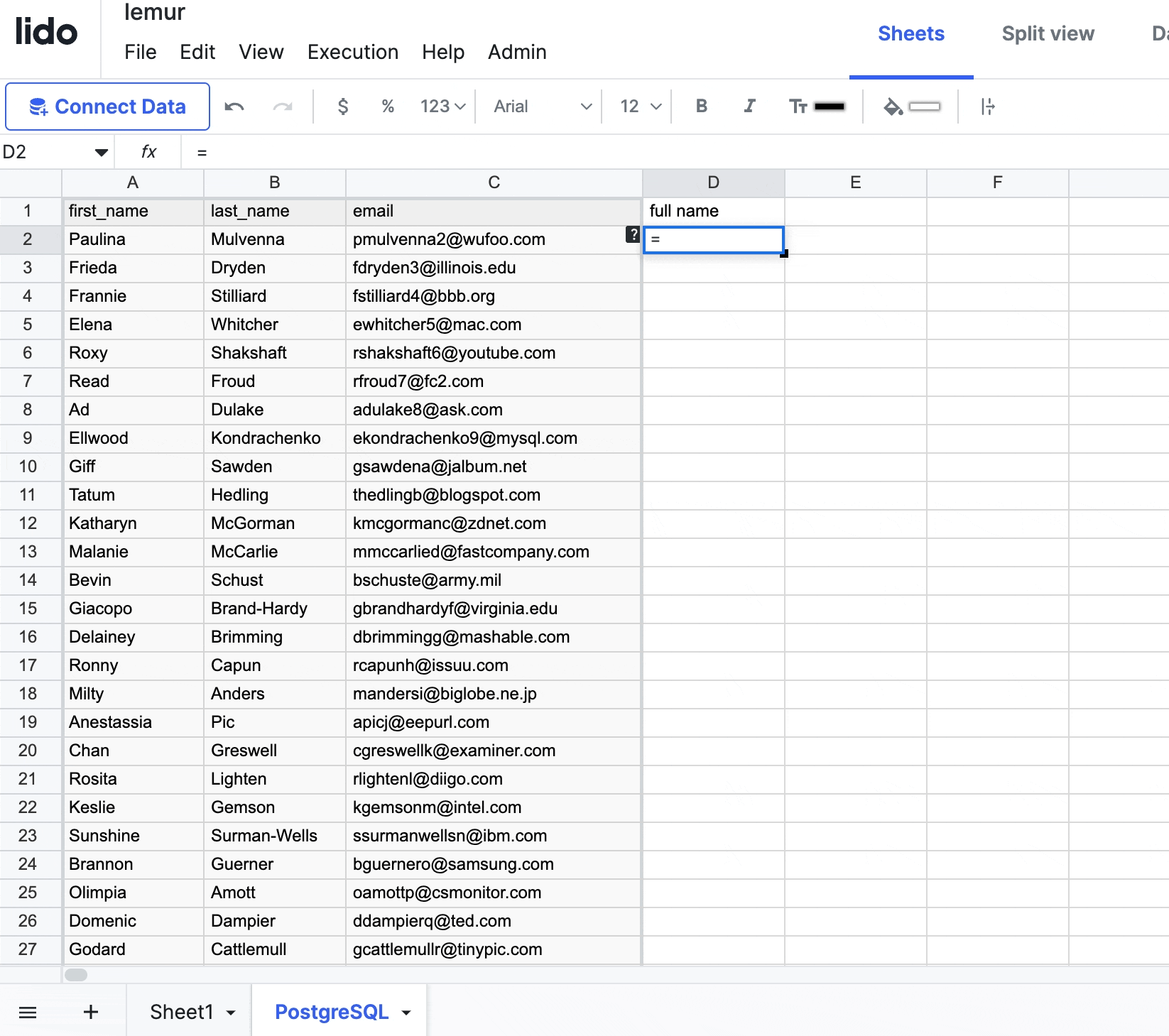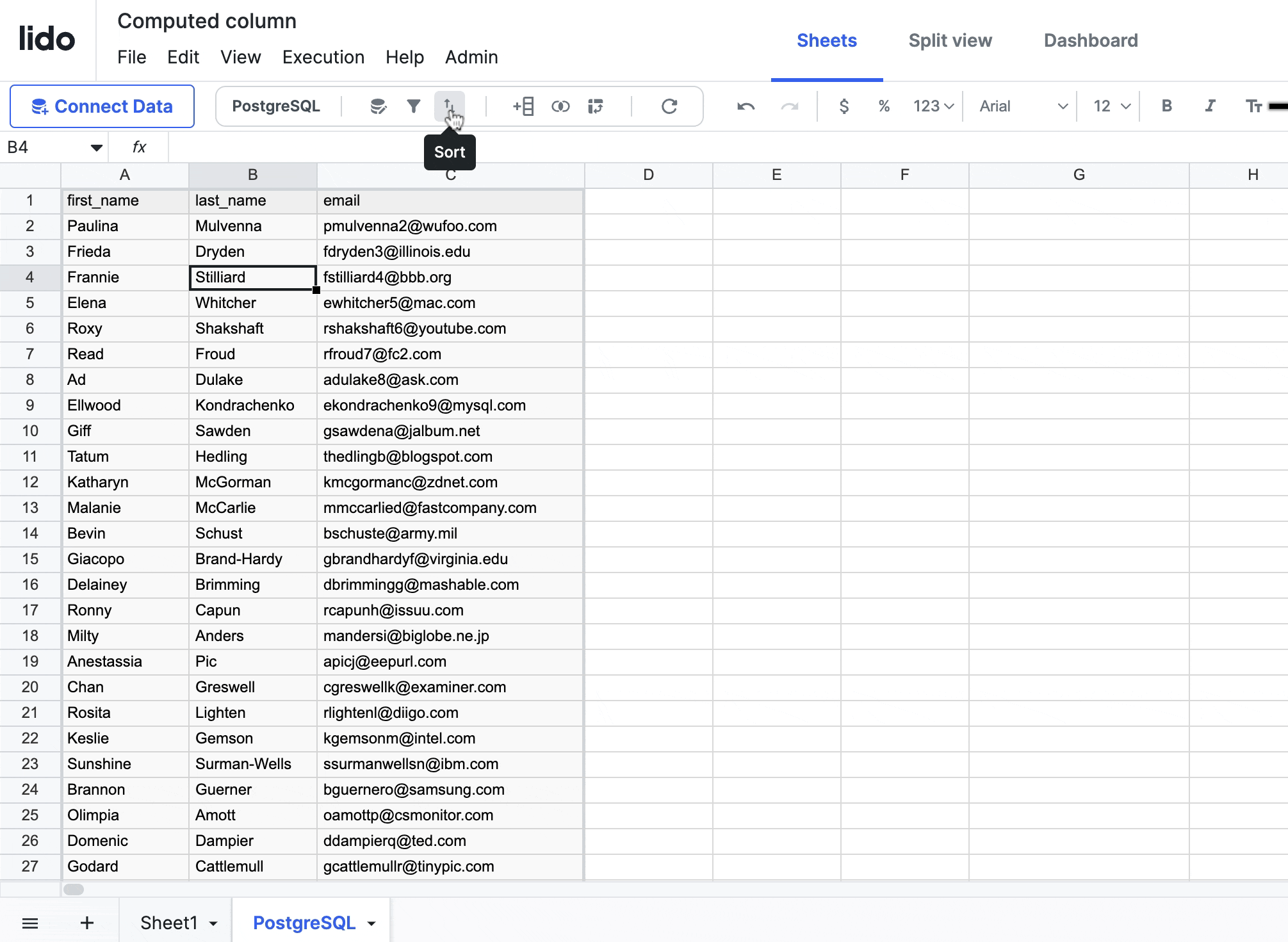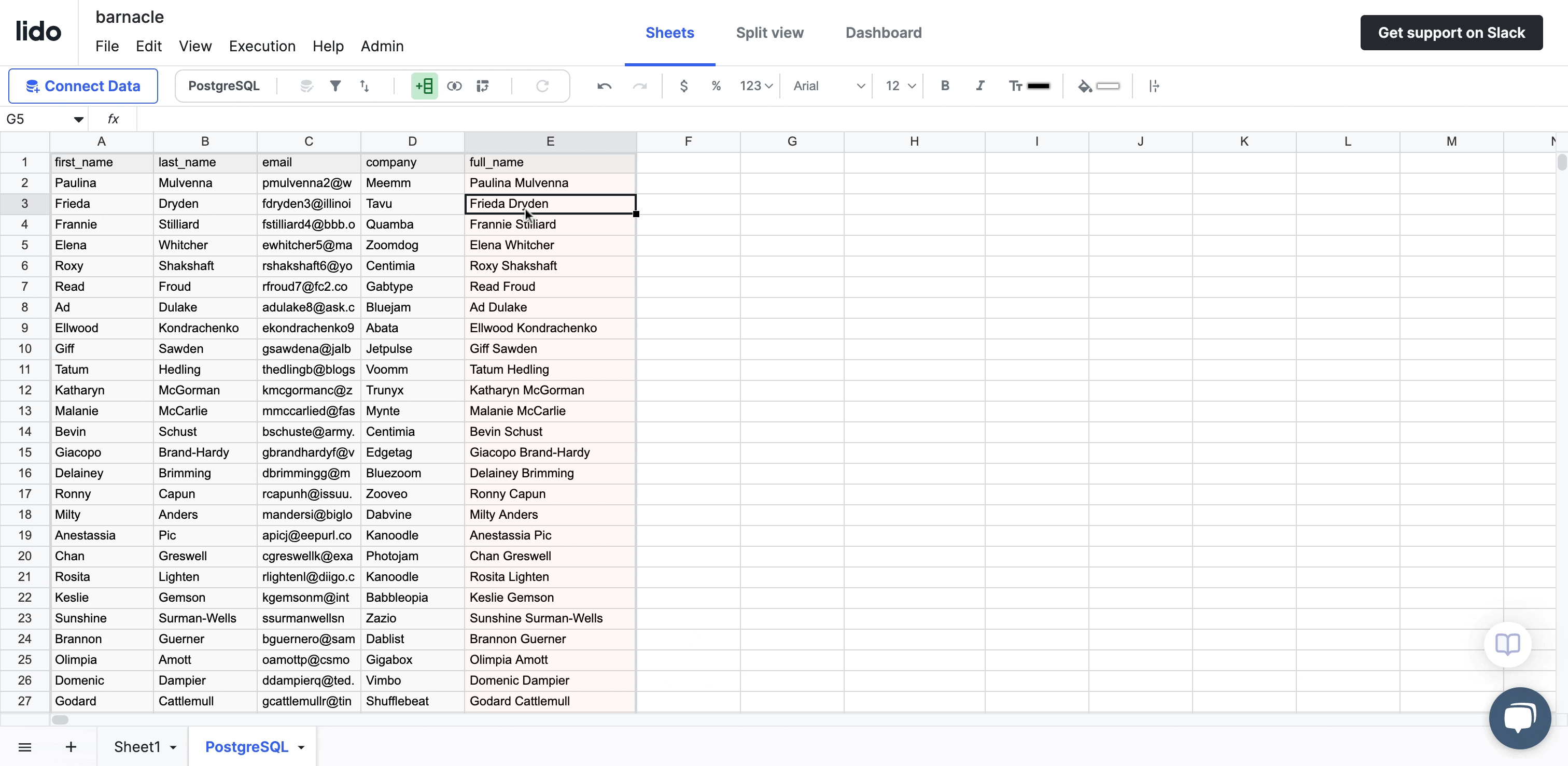# Computed columns

Learn how to use computed columns in Lido

A computed column is type of column that can be added to a spreadsheet table. The values in the computed column are determined by a formula that is applied to every row in the table. If the length of the table changes (e.g. if more rows get added to a datasource) then the computed column will update automatically to reflect those changes.

Computed columns are incredibly useful for tasks such as data normalization, complex calculations, transformations, or data manipulation.

# How to add a computed column

There are two ways to create computed columns in Lido.

Add the formula for your computed column in a cell directly next to the table where you want the column to be added### From the toolbar

When your cursor is inside of the table, click on the computed column icon in the toolbar to open the computed column modal.# Editing computed columns

The easiest way to edit or rename a computed column is through the toolbar.